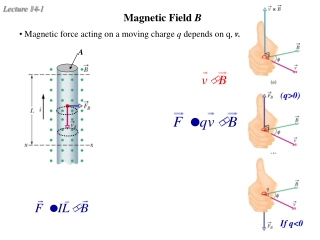DownloadDownload PresentationMagnetic Field B

# Magnetic Field B

Télécharger la présentation## Magnetic Field B

- - - - - - - - - - - - - - - - - - - - - - - - - - - E N D - - - - - - - - - - - - - - - - - - - - - - - - - - -
##### Presentation Transcript

1. A If q<0 Magnetic Field B • Magnetic force acting on a moving charge q depends on q, v. (q>0)

2. EH EH Hall Effect • A conducting strip in crossed E and B fields • Applied E along the strip leads to a charge buildup on the sides of the strip and thus an electric field EH develops  to both applied E and B. • Determines the sign and number of carriers. • Measures B.

3. Hall voltage EH EH for a given current I and n Carrier Sign and Density from Hall Effect Sign and density of charge carrier is determined at equilibrium and

4. increases as v does Cyclotron • "Magnetic Resonance Accelerator" • "Dees" in constant magnetic field B • Alternating voltage V is applied between the Dees at the orbital frequency f: • Particle will acquire an additional kinetic energy T = qV each time it crosses the gap (ie twice per revolution.. E=0 in Dees!). problems  synchrotron

5. Synchrotron R is the same since B increases as v does

6. Also non-uniform B magnetic bottle Van Allen belts More complicated situations? v is not perpendicular to B helical motion (spiral)

7. Polar Light High energy particles leaked out of the belt and interact with the earth atmosphere.

8. Warm-up An electron (charge -e) comes horizontally into a region of perpendicularly crossed, uniform E and B fields as shown. In this region, it deflects upward as shown. What can you do to change the path so it remains horizontal through the region? Increase E Increase B Turn B off Turn E off Nothing http://canu.ucalgary.ca/map/content/force/elcrmagn/simulate/magnetic/applet.html http://canu.ucalgary.ca/map/content/force/elcrmagn/simulate/exb_thomson/applet.html

9. closed loop Magnetic Force on a Current Loop Force on closedloop current in uniform B? • Force on top path cancels force on bottom path (F = IBL) • Force on right path cancels force on left path. (F = IBL) Uniform B exerts no net force on closed current loop.

10. Definition of torque: abut a chosen point Magnetic Torque on a Current Loop • If B field is  to plane of loop, the net torque on loop is also 0. B • If B is not , there is net torque.

11. area of loop Calculation of Torque • Suppose the coil has width b (the side we see) and length a (into the screen). The torque about the center is given by: • Define magnetic dipole moment by where n is normal to the loop with RHR along I.

12. A thin non-conducting disk of mass m and uniform surface charge density  rotates with angular velocity  as shown. What is the magnetic moment? mag. moment of the ring shown: dI Example of Magnetic Moment Calculation

13. Work must be done to change the orientation of a dipole (current loop) in the presence of a magnetic field. Potential Energy of Dipole B x • Define a potential energy U (with zero at position of max torque) corresponding to this work. . q Þ Therefore, Þ Þ

14. m x B B B t= mB m m x x X positive work Potential Energy of Dipole Illustrated t= 0 U = -mB t= 0 U =mB U = 0 max torque min. energy max. energy negative work (by YOU)

15. PHYS241 - Quiz A An electron (charge e) comes horizontally into a region of perpendicularly crossed, uniform E and B fields as shown. In this region, it is deflected upward as shown. What can you do to change the path so it deflects downward instead through the region? a. Increase E b. Turn B off c. Decrease E d. Slow down the electron e. None of the above

16. PHYS241 - Quiz B A proton (charge +e) comes horizontally into a region of perpendicularly crossed, uniform E and B fields as shown. In this region, it goes straight without deflection. What can you do to change the path so it deflects upward through the region? a. Increase E b. Increase B c. Turn B off d. Slow down the proton e. None of the above

17. PHYS241 - Quiz C A proton (charge +e) comes horizontally into a region of perpendicularly crossed, uniform E and B fields as shown. In this region, it deflects downward as shown. What can you do to change the path so it remains horizontal through the region? a. Increase E b. Turn B off c. Turn E off d. Slow down the electron e. Increase B